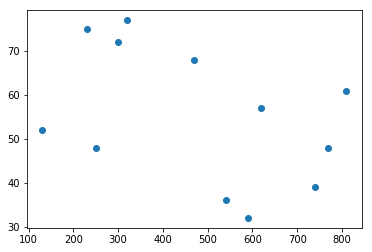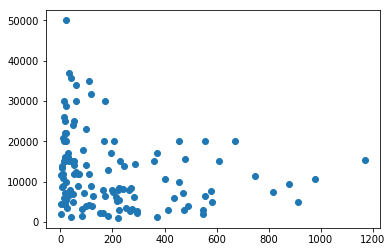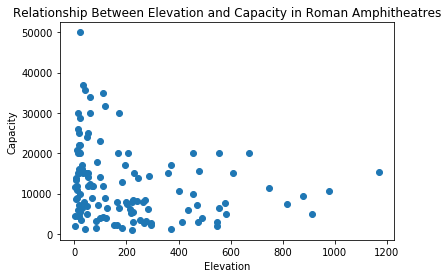# Analyzing and Visualizing Data From a Database¶

In the previous notebook you combined some csv tables into a SQLite database. You learned how to write various queries to examine the data. In this notebook, you'll write a query into a 'dataframe', a table that you can then manipulate or visualize.

NB you need to have created the roman.db in the first notebook (part one of that notebook) before you can progress any further here.

In :
# We tell python to use the sqlite3 and pandas modules

import sqlite3
import pandas as pd

# we connect our database
db = sqlite3.connect('roman.db')

In :
# now we run a query; but unlike in the previous notebook, we'll put all of the SQL commands on a single line
# the line below defines a dataframe, 'df', and uses the pandas command pd.read_sql_query to run a query on the database connection we defined above, db.

df = pd.read_sql_query("SELECT id, elevation, capacity FROM amphi ORDER BY elevation DESC", db)

In :
# let's check that we got the info. we call the first five rows of the dataframe like so:

df[0:10]

Out:
id elevation capacity
0 lambaesisAmphitheater 1170 15400
1 albaFucensAmphitheater 977 10720
2 mactarisAmphitheater 911 5000
3 tebessaAmphitheater 877 9400
4 leonAmphitheater 846
5 bostraAmphitheater 845
6 segobrigaAmphitheater 817 7500
7 siccaVeneriaAmphitheater 748 11300
8 sanBenedettoDeiMarsiAmphitheater 683
9 sanVittorinoAmphitheater 672 20000
In :
# those empty cells are problematic
# Pandas expects missing values to be coded NaN rather than just be empty cells.

# We need to check to see whether or not our data is actually numeric

from pandas.api.types import is_string_dtype
from pandas.api.types import is_numeric_dtype

# is the data of the type 'string'?
is_string_dtype(df['capacity'])

Out:
True
In :
# is the data of the type 'numeric'? We need it to be numeric in order to plot it.
is_numeric_dtype(df['capacity'])

Out:
False
In :
# We covert the column to the numeric data type.
df['capacity'] = df['capacity'].apply(pd.to_numeric, errors='coerce')

In :
# checking to see
is_string_dtype(df['capacity'])

Out:
False
In :
# compare the results here with the first time we looked. What's different?
df[0:5]

Out:
id elevation capacity
0 lambaesisAmphitheater 1170 15400.0
1 albaFucensAmphitheater 977 10720.0
2 mactarisAmphitheater 911 5000.0
3 tebessaAmphitheater 877 9400.0
4 leonAmphitheater 846 NaN
In :
# Now we can begin to plot data.
# to plot things with pandas, we also need a package called matplotlib
%matplotlib inline
import matplotlib.pyplot as plt

In :
# here's how we make a simple scatter plot
# you will be able to find lots of documentation online for the various kinds of things matplotlib can do.

X = [590,540,740,130,810,300,320,230,470,620,770,250]
Y = [32,36,39,52,61,72,77,75,68,57,48,48]

plt.scatter(X,Y)
plt.show()In :
# Let's plot elevation against capacity. There are many ways of doing it; here we show two that both produce the same result. Uncomment and comment out (remove or add the #) to try one versus the other
df.plot.scatter('elevation', 'capacity')
# plt.scatter(df['elevation'], df['capacity'])
plt.show()In :
# Let's make the plot pretty

plt.scatter(df['elevation'], df['capacity'])
plt.title('Relationship Between Elevation and Capacity in Roman Amphitheatres')

plt.xlabel('Elevation')
plt.ylabel('Capacity')

Out:
Text(0,0.5,'Capacity')In :
# let's print the plot to raster and to vector image